# Resources tagged with: Geometric sequences

Filter by: Content type:
Age range:
Challenge level:

### There are 20 results

Broad Topics > Patterns, Sequences and Structure > Geometric sequences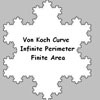### Von Koch Curve

##### Age 16 to 18Challenge Level

Make a poster using equilateral triangles with sides 27, 9, 3 and 1 units assembled as stage 3 of the Von Koch fractal. Investigate areas & lengths when you repeat a process infinitely often.### Sierpinski Triangle

##### Age 16 to 18Challenge Level

What is the total area of the triangles remaining in the nth stage of constructing a Sierpinski Triangle? Work out the dimension of this fractal.### Golden Fibs

##### Age 16 to 18Challenge Level

When is a Fibonacci sequence also a geometric sequence? When the ratio of successive terms is the golden ratio!##### Age 16 to 18Challenge Level

A circle is inscribed in an equilateral triangle. Smaller circles touch it and the sides of the triangle, the process continuing indefinitely. What is the sum of the areas of all the circles?### Tiny Nines

##### Age 14 to 16Challenge Level

What do you notice about these families of recurring decimals?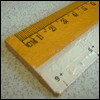### Ruler

##### Age 16 to 18Challenge Level

The interval 0 - 1 is marked into halves, quarters, eighths ... etc. Vertical lines are drawn at these points, heights depending on positions. What happens as this process goes on indefinitely?### Vanishing Point

##### Age 14 to 18Challenge Level

How can visual patterns be used to prove sums of series?### Converging Product

##### Age 16 to 18Challenge Level

In the limit you get the sum of an infinite geometric series. What about an infinite product (1+x)(1+x^2)(1+x^4)... ?### Binary Squares

##### Age 16 to 18Challenge Level

If a number N is expressed in binary by using only 'ones,' what can you say about its square (in binary)?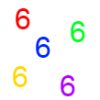### Clickety Click

##### Age 16 to 18 ShortChallenge Level

What is the sum of: 6 + 66 + 666 + 6666 ............+ 666666666...6 where there are n sixes in the last term?### Sum the Series

##### Age 16 to 18

This article by Alex Goodwin, age 18 of Madras College, St Andrews describes how to find the sum of 1 + 22 + 333 + 4444 + ... to n terms.### Generally Geometric

##### Age 16 to 18Challenge Level

Generalise the sum of a GP by using derivatives to make the coefficients into powers of the natural numbers.### Production Equation

##### Age 16 to 18Challenge Level

Each week a company produces X units and sells p per cent of its stock. How should the company plan its warehouse space?### Squaring the Circle and Circling the Square

##### Age 14 to 16Challenge Level

If you continue the pattern, can you predict what each of the following areas will be? Try to explain your prediction.### Sixty-seven Squared

##### Age 16 to 18Challenge Level

Evaluate these powers of 67. What do you notice? Can you convince someone what the answer would be to (a million sixes followed by a 7) squared?### Geometric Parabola

##### Age 14 to 16Challenge Level

Explore what happens when you draw graphs of quadratic equations with coefficients based on a geometric sequence.### Double Trouble

##### Age 14 to 16Challenge Level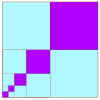### Summing Geometric Progressions

##### Age 14 to 18Challenge Level

Watch the video to see how to sum the sequence. Can you adapt the method to sum other sequences?### Proof Sorter - Geometric Sequence

##### Age 16 to 18Challenge Level

Can you correctly order the steps in the proof of the formula for the sum of the first n terms in a geometric sequence?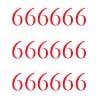### Clickety Click and All the Sixes

##### Age 16 to 18Challenge Level

What is the sum of: 6 + 66 + 666 + 6666 ............+ 666666666...6 where there are n sixes in the last term?